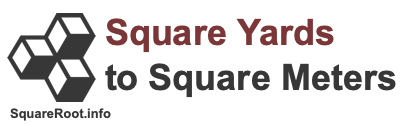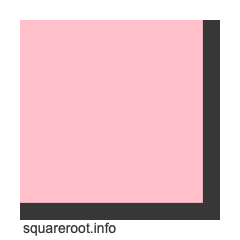Square Yards to Square Meters ConverterHere we will explain and show you how to convert square yards to square meters. Before we continue, note that square yards to square meters is also known as sq yards to sq meters, sqyd to sqm, yd2 to m2, and yd² to m².

To create a formula to convert square yards to square meters, we start with the fact that one yard equals 0.9144 meters. Therefore, this formula is true:

yards × 0.9144 = meters

However, we are dealing with square yards and square meters, which means yards and meters to the 2nd power. Thus, we take both sides of the formula above to the 2nd power to get this result:

yards² × 0.9144² = meters²
yards² × 0.83612736 = meters²
yd² × 0.83612736 = m²

Now we know that one square yard is equal to 0.83612736 square meters, and that the formula to convert square yards to square meters is as follows:

yd² × 0.83612736 = m²

Below is an illustration showing you how one square meter fits into one square yard. The cut out in pink is how one square meter fits into the entire square yard. One square meter is about 83.61 percent of a square yard.To convert square yards into square meters using our formula above, please enter your number of square yards below:

Convert  square yards to square meters.

Below are some examples of what our Square Yards to Square Meters Converter can convert and calculate for you.

10 square yards to square meters

25 square yards to square meters

50 square yards to square meters

100 square yards to square meters